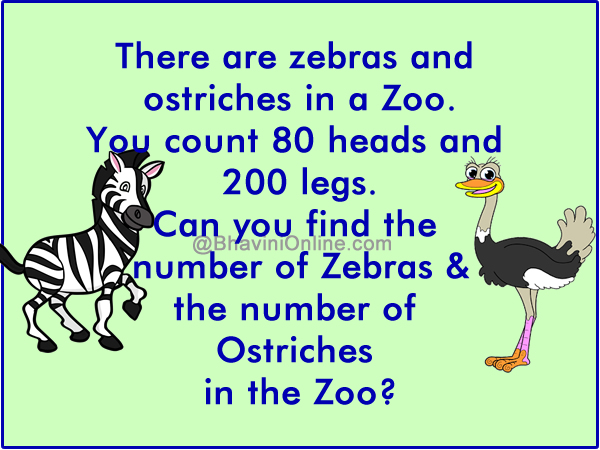# Zoo Riddle: Find the Number Of Zebras And Ostriches

You visit a zoo in Africa.

There are zebras and ostriches in this Zoo.

You count 80 heads and 200 legs.

Can you find the number of Zebras and the number of Ostriches in the Zoo?To solve this riddle we will have to form equations.

Let the number of Zebras be Z &

the number of Ostriches be O.

Since there are 80 heads we can write;

Z + O = 80.—————————(1)

Since we know there are 200 legs  in all and Ostrich has 2 legs whereas zebra has 4 legs we can write the following;

4Z + 2O = 200.———————-(2)

Multiplying 2 to equation (1) and then subtracting it from equation (2) we get;

4Z + 2O = 200
– 2Z – 2O = 160
2Z   = 40

Z = 20.

Substituting value of Z in (1);

60 + O = 80

Therefore;

O = 60

The number of Ostriches = 60 &

The number of Zebras = 20